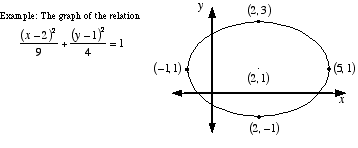index: click on a letter A B C D E F G H I J K L M N O P Q R S T U V W X Y Z A to Z index index: subject areas numbers & symbols sets, logic, proofs geometry algebra trigonometry advanced algebra & pre-calculus calculus advanced topics probability & statistics real world applications multimedia entrieswww.mathwords.com about mathwords website feedback

 Relation For example, {(1, 2), (3, 4), (1, a), (5, r)} is a relation. So is the set {(x, y): y = x2}; this is the set of all ordered pairs (x, y) for which y = x2.See also